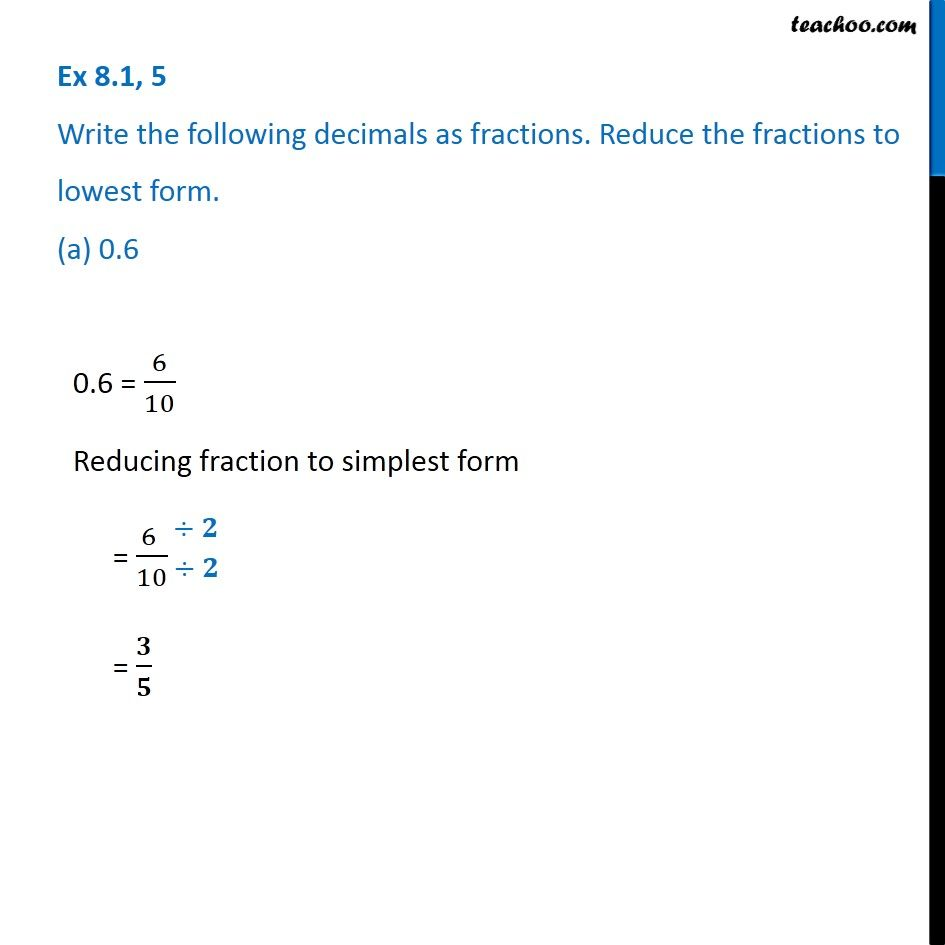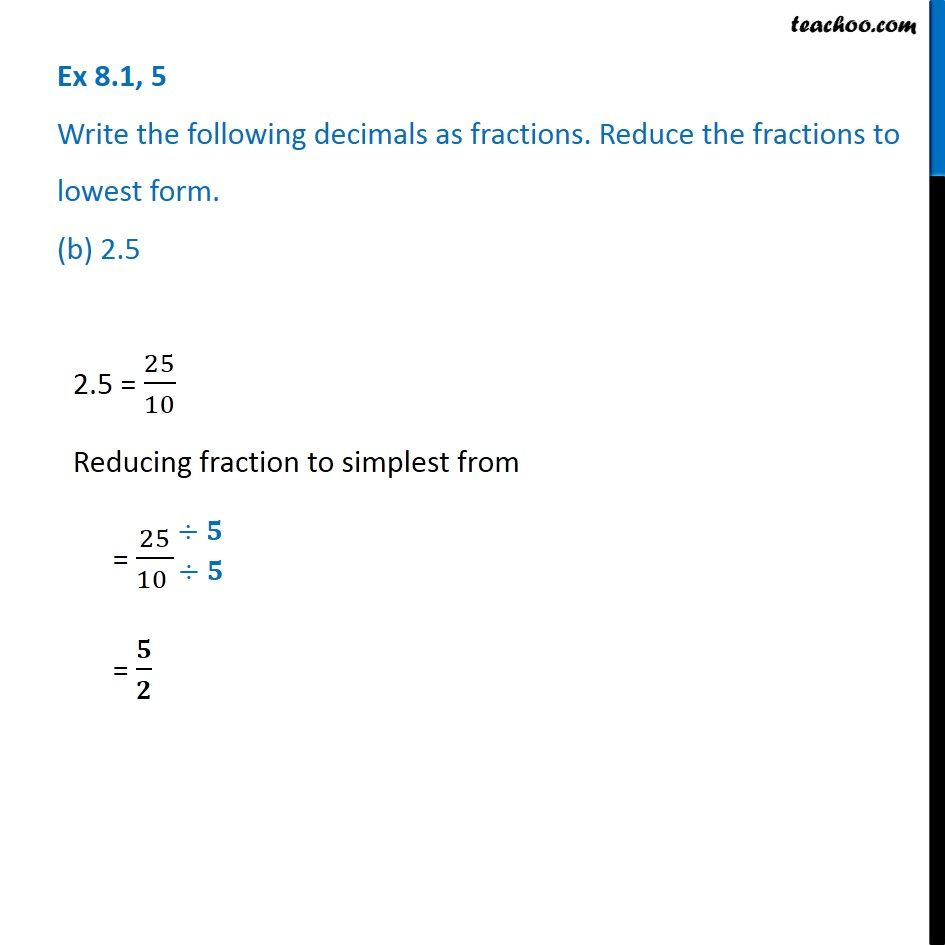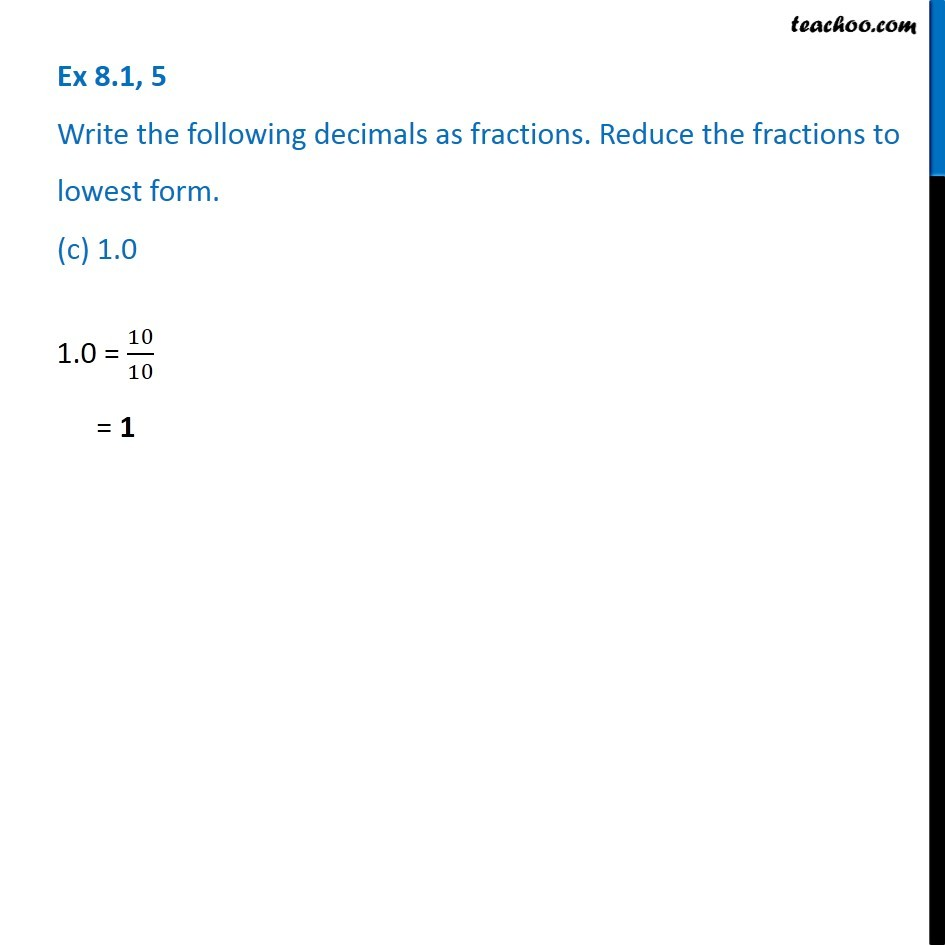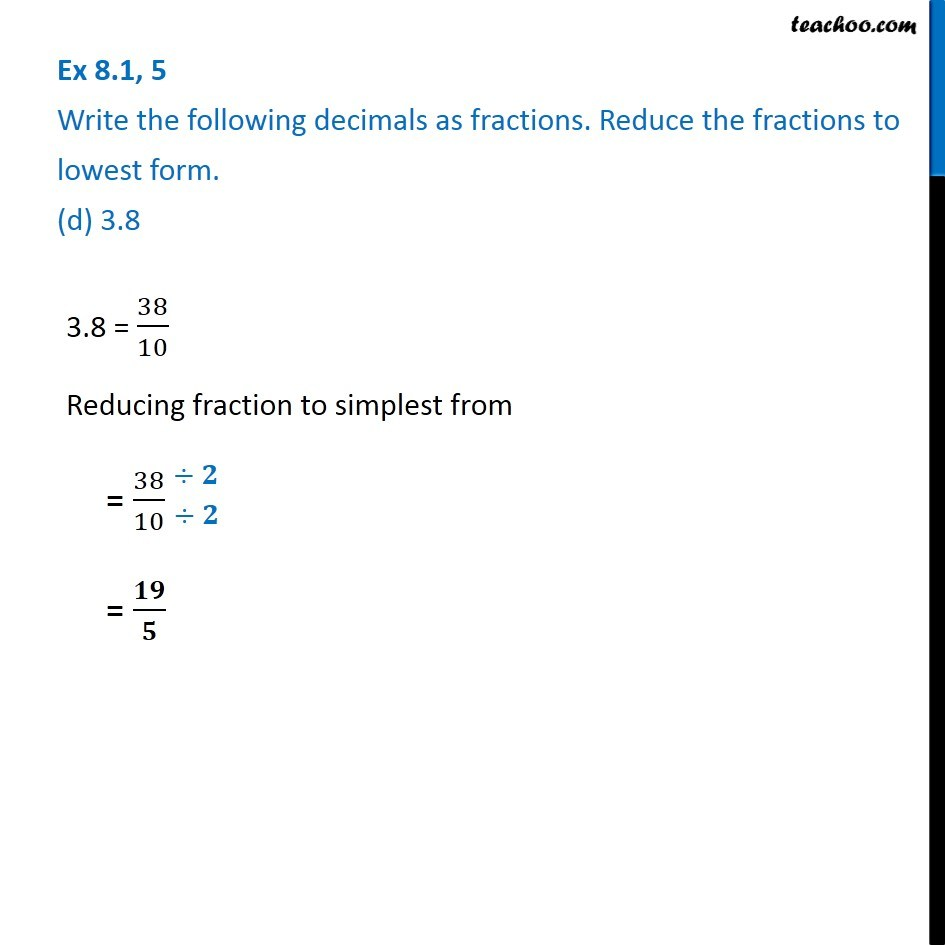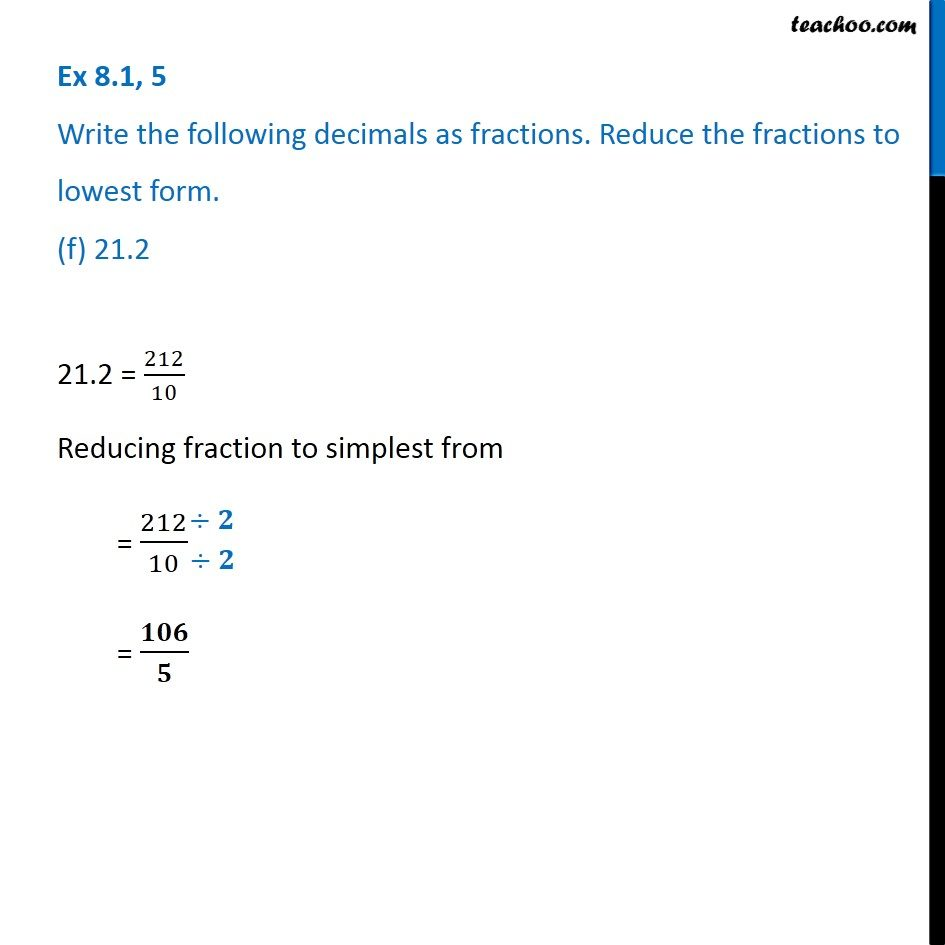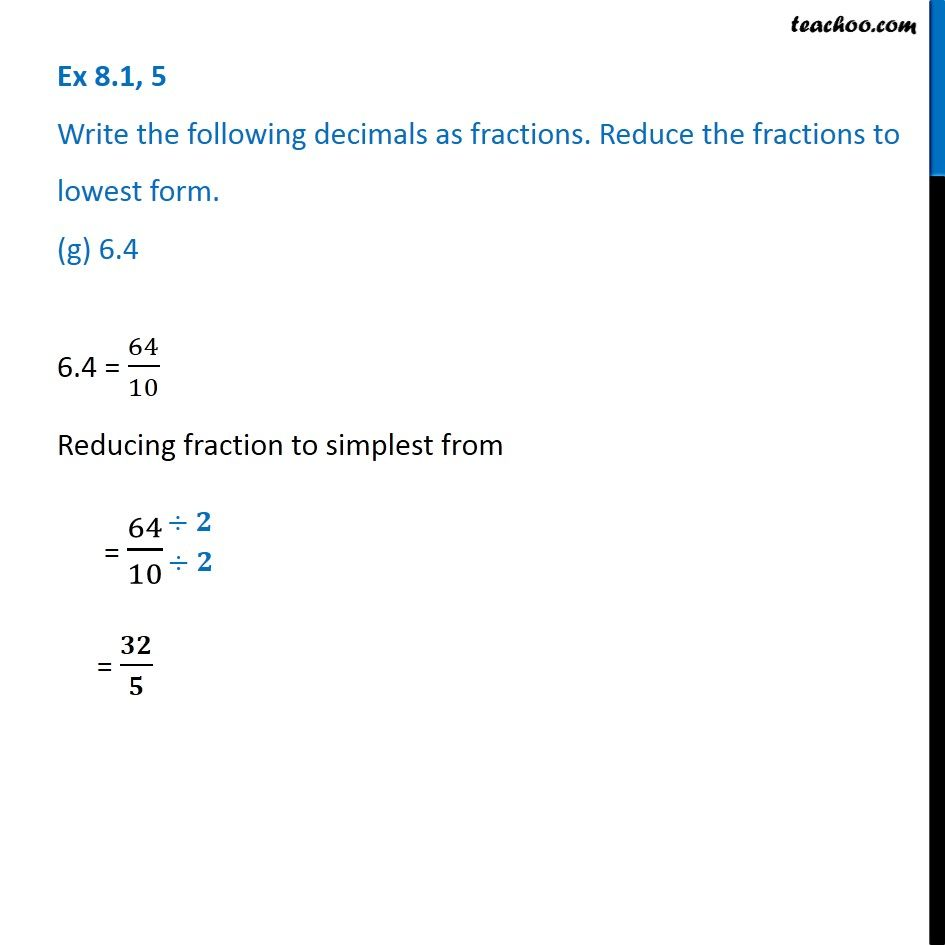1. Chapter 8 Class 6 Decimals
2. Serial order wise
3. Ex 8.1

Transcript

Ex 8.1, 5 Write the following decimals as fractions. Reduce the fractions to lowest form. (a) 0.6 0.6 = 6/10 Reducing fraction to simplest form = (6 )/10 = 𝟑/𝟓 Ex 8.1, 5 Write the following decimals as fractions. Reduce the fractions to lowest form. (b) 2.5 2.5 = 25/10 Reducing fraction to simplest from = 25/(10 ) = 𝟓/𝟐 Ex 8.1, 5 Write the following decimals as fractions. Reduce the fractions to lowest form. (c) 1.0 1.0 = 10/10 = 1 Ex 8.1, 5 Write the following decimals as fractions. Reduce the fractions to lowest form. (d) 3.8 3.8 = 38/10 Reducing fraction to simplest from = 38/10 = 𝟏𝟗/𝟓 Ex 8.1, 5 Write the following decimals as fractions. Reduce the fractions to lowest form. (e) 13.7 13.7 = 137/10 This fraction cannot be further reduced Ex 8.1, 5 Write the following decimals as fractions. Reduce the fractions to lowest form. (f) 21.2 21.2 = 212/10 Reducing fraction to simplest from = 212/10 = 𝟏𝟎𝟔/𝟓 Ex 8.1, 5 Write the following decimals as fractions. Reduce the fractions to lowest form. (g) 6.4 6.4 = 64/10 Reducing fraction to simplest from = 64/10 = 𝟑𝟐/𝟓

Ex 8.1

Chapter 8 Class 6 Decimals
Serial order wise